# centrality

## 语法

``C = centrality(G,type)``
``C = centrality(___,Name,Value)``

## 说明

``C = centrality(G,type)` 为图中的每个节点计算由 `type` 指定的节点中心性。 `

``C = centrality(___,Name,Value)` 使用一个或多个名称-值对组参数指定的其他选项。例如，`centrality(G,'closeness','Cost',c)` 指定遍历每条边的成本。`

## 示例

```s = [1 1 2 2 3 3 3 4 5]; t = [2 5 3 4 4 5 6 1 1]; names = {'http://www.example.com/alpha', 'http://www.example.com/beta', ... 'http://www.example.com/gamma', 'http://www.example.com/delta', ... 'http://www.example.com/epsilon', 'http://www.example.com/zeta'}; G = digraph(s,t,[],names); plot(G,'NodeLabel',{'alpha','beta','gamma','delta','epsilon','zeta'})```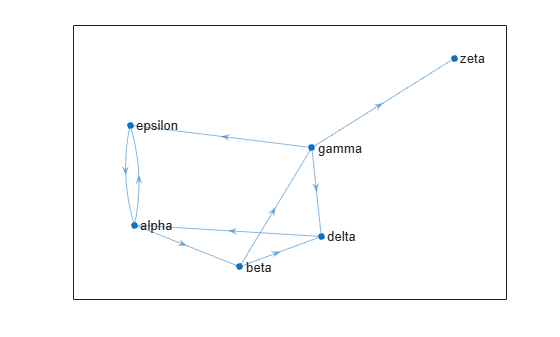`pg_ranks = centrality(G,'pagerank')`
```pg_ranks = 6×1 0.3210 0.1706 0.1066 0.1368 0.2008 0.0643 ```
```G.Nodes.PageRank = pg_ranks; G.Nodes```
```ans=6×2 table Name PageRank __________________________________ ________ {'http://www.example.com/alpha' } 0.32098 {'http://www.example.com/beta' } 0.17057 {'http://www.example.com/gamma' } 0.10657 {'http://www.example.com/delta' } 0.13678 {'http://www.example.com/epsilon'} 0.20078 {'http://www.example.com/zeta' } 0.06432 ```

```hub_ranks = centrality(G,'hubs'); auth_ranks = centrality(G,'authorities'); G.Nodes.Hubs = hub_ranks; G.Nodes.Authorities = auth_ranks;```
`G.Nodes`
```ans=6×4 table Name PageRank Hubs Authorities __________________________________ ________ __________ ___________ {'http://www.example.com/alpha' } 0.32098 0.24995 7.3237e-05 {'http://www.example.com/beta' } 0.17057 0.24995 0.099993 {'http://www.example.com/gamma' } 0.10657 0.49991 0.099993 {'http://www.example.com/delta' } 0.13678 9.1536e-05 0.29998 {'http://www.example.com/epsilon'} 0.20078 9.1536e-05 0.29998 {'http://www.example.com/zeta' } 0.06432 0 0.19999 ```

```A = sprand(1000,1000,0.15); A = A + A'; G = graph(A,'omitselfloops'); p = plot(G,'Layout','force','EdgeAlpha',0.005,'NodeColor','r');```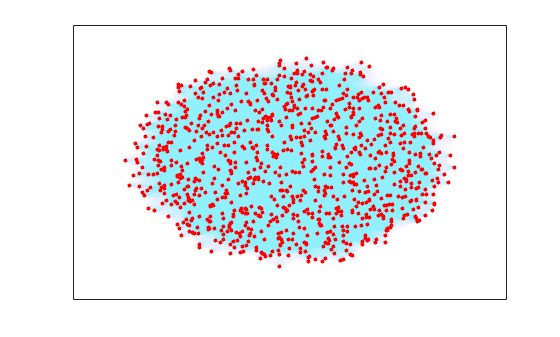`deg_ranks = centrality(G,'degree','Importance',G.Edges.Weight);`

```edges = linspace(min(deg_ranks),max(deg_ranks),7); bins = discretize(deg_ranks,edges);```

`p.MarkerSize = bins;`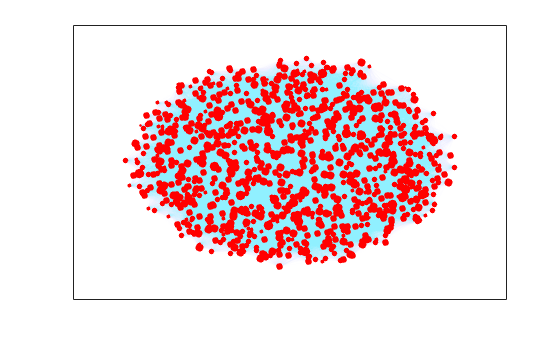```load minnesota.mat xy = [G.Nodes.XCoord G.Nodes.YCoord];```

```[s,t] = findedge(G); G.Edges.Weight = hypot(xy(s,1)-xy(t,1), xy(s,2)-xy(t,2));```

```p = plot(G,'XData',xy(:,1),'YData',xy(:,2),'MarkerSize',5); title('Minnesota Road Network')```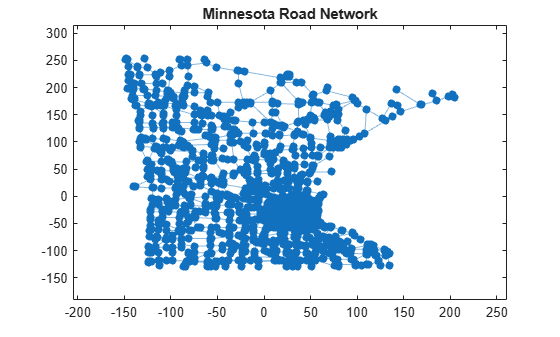```ucc = centrality(G,'closeness'); p.NodeCData = ucc; colormap jet colorbar title('Closeness Centrality Scores - Unweighted')```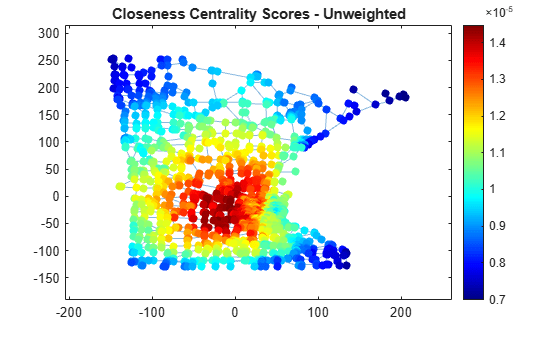```wcc = centrality(G,'closeness','Cost',G.Edges.Weight); p.NodeCData = wcc; title('Closeness Centrality Scores - Weighted')```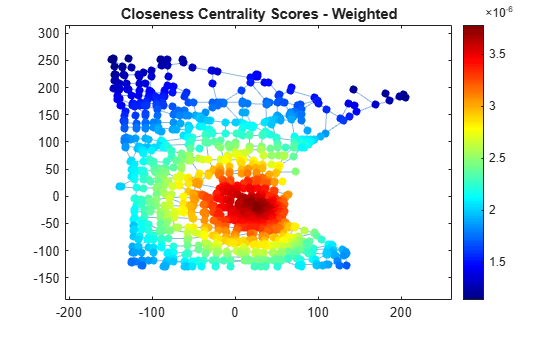```wbc = centrality(G,'betweenness','Cost',G.Edges.Weight); n = numnodes(G); p.NodeCData = 2*wbc./((n-2)*(n-1)); colormap(flip(autumn,1)); title('Betweenness Centrality Scores - Weighted')```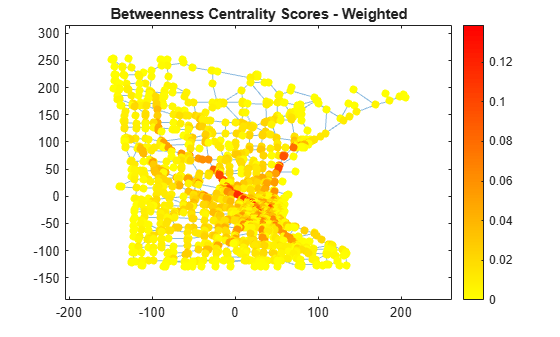## 输入参数

`'degree'`

`'degree'``'outdegree'``'indegree'` 中心性类型基于每个节点连接的边数：

• `'degree'` - 每个节点连接的边数。一个自环记为与节点连接的两条边。

• `'indegree'` - 每个节点的入向边数。一个自环记为一条入向边。

• `'outdegree'` - 每个节点的出向边数。一个自环记为一条出向边。

`'Importance'`

`'indegree'`

`'outdegree'`

`'closeness'`

`'closeness'``'incloseness'``'outcloseness'` 中心性类型使用图中从一个节点到其他所有节点的距离的总和的倒数。如果并非所有节点都可达，则节点 `i` 的中心性为：

`$c\left(i\right)={\left(\frac{{A}_{\text{\hspace{0.17em}}i}}{N-1}\right)}^{2}\frac{1}{{C}_{i}}\text{\hspace{0.17em}}.$`

Ai 是可从节点 `i` 到达的节点数（不计 `i`），N 是 `G` 中的节点数，Ci 是从节点 `i` 到所有可到达节点之间的距离之和。

• 如果从节点 `i` 不能到达任何节点，则 `c(i)` 为零。

• 对于 `'incloseness'`，距离测量值是从所有节点到节点 `i`

• `'Cost'` 边权重指定边的长度。

`'Cost'`

`'incloseness'`

`'outcloseness'`

`'betweenness'`

`'betweenness'` 中心性类型衡量每个图节点出现在图中两个节点之间的最短路径上的频率。由于两个图节点 `s``t` 之间可能存在多个最短路径，因此节点 `u` 的中心性为：

`$c\left(u\right)=\sum _{s,t\ne u}\frac{{n}_{st}\left(u\right)}{{N}_{st}}\text{\hspace{0.17em}}.$`

${n}_{st}\left(u\right)$ 是从 `s``t` 且穿过节点 `u` 的最短路径数，${N}_{st}$ 是从 `s``t` 的最短路径总数。

• 如果是无向图，则从 `s``t` 与从 `t``s` 的路径只记为一条路径（将公式除以 2）。

• `'Cost'` 边权重指定边的长度，帮助确定节点 `s``t` 之间的最短路径。

`'Cost'`

`'pagerank'`

`'pagerank'` 中心性类型来自网络的随机游走。在图中的每个节点处，按照概率 `'FollowProbability'` 从当前节点的后继节点集（无向图中的相邻节点）中选择下一个节点。否则，当一个节点没有后继节点时，将从所有节点中选择下一个节点。中心性得分是指随机游走期间在每个节点上花费的平均时间。

• 如果某个节点存在自环，则算法可能会遍历该循环。因此，自环会增加所连接节点的 PageRank 中心性得分。

• 在相同的两个节点之间具有多条边的多重图中，具有多条边的节点被选择的可能性更大。

• `'Importance'` 边权重影响算法选择后继节点的方式。重要性越高的节点，被选择的可能性越大。

`'Importance'`

`'FollowProbability'`

`'Tolerance'`

`'MaxIterations'`

`'eigenvector'`

`'eigenvector'` 中心性类型使用与图邻接矩阵的最大特征值对应的特征向量。得分经过归一化，因此所有中心性得分的总和等于 1。

• 如果有多个未连通分量，算法将分别计算每个分量的特征向量中心性，然后根据图节点在该分量中的百分比来折算分数。

• 未连接节点的中心性得分为 `1/numnodes(G)`

• 指定 `'Importance'` 边权重以在计算时使用加权的邻接矩阵。

`'Importance'`

`'Tolerance'`

`'MaxIterations'`

`'hubs'`

`'authorities'`

`'hubs'``'authorities'` 中心性得分是两个链接的递归中心性测量值。一个节点的枢纽得分是其所有后继节点的权威得分之和。同样，权威得分是其所有前趋节点的枢纽得分之和。所有枢纽得分之和等于 1，所有权威得分之和也等于 1。

• 这些得分可理解为与邻接矩阵的最大奇异值对应的左侧（枢纽）和右侧（权威）奇异向量。

• 未连接节点的中心性得分为 `1/numnodes(G)`

• 指定 `'Importance'` 边权重以使用加权和，而不是将所有后继/前趋节点的得分简单相加。这相当于使用加权的邻接矩阵的奇异向量。

• 如果有多个未连通分量（在弱连通意义上），该算法将分别计算每个分量的枢纽得分和权威得分。然后根据图节点在该分量中的百分比来折算分数，从而使总和依然为 1。

`'Importance'`

`'Tolerance'`

`'MaxIterations'`

`centrality` 函数假定所有边权重都等于 1。要更改此设置，请指定边权重与 `'Cost'``'Importance'` 名称-值对组一起使用。

### 名称-值参数

• 对于 `'closeness'``'outcloseness'``'incloseness'` 中心性类型，边成本必须为非负值。

• 对于 `'betweenness'` 中心性类型，边成本必须为正值。

• 旅程长度

• 差旅时间

• 机票成本

`'Cost'` 仅适用于 `'closeness'``'outcloseness'``'incloseness'``'betweenness'` 中心性类型。

`'FollowProbability'` 仅适用于 `'pagerank'` 中心性类型。

• 每天的旅行人数

• 链接的点击次数

• 发表的论文总数

`'Importance'` 仅适用于 `'degree'``'outdegree'``'indegree'``'pagerank'``'eigenvector'``'hubs'``'authorities'` 中心性类型。

`'MaxIterations'` 仅适用于 `'pagerank'``'eigenvector'``'hubs'``'authorities'` 中心性类型。

`'Tolerance'` 仅适用于 `'pagerank'``'eigenvector'``'hubs'``'authorities'` 中心性类型。